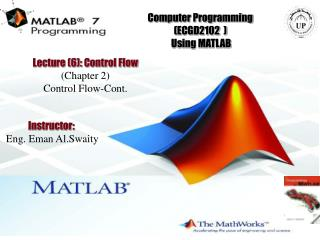Download PresentationComputer Programming (ECGD2102 ) Using MATLABComputer Programming (ECGD2102 ) Using MATLAB - PowerPoint PPT Presentation

Download PresentationComputer Programming (ECGD2102 ) Using MATLAB
An Image/Link below is provided (as is) to download presentation

Download Policy: Content on the Website is provided to you AS IS for your information and personal use and may not be sold / licensed / shared on other websites without getting consent from its author. While downloading, if for some reason you are not able to download a presentation, the publisher may have deleted the file from their server.

- - - - - - - - - - - - - - - - - - - - - - - - - - - E N D - - - - - - - - - - - - - - - - - - - - - - - - - - -
Presentation Transcript

1. Computer Programming(ECGD2102 ) Using MATLAB Lecture (6): Control Flow (Chapter 2) Control Flow-Cont. Instructor: Eng. Eman Al.Swaity

2. Objectives (CHAPTER (2):Control Flow) At the end of this lesson, you will be able to understand: • Study the different methods for control flow in MATLAB • Introduce and use for-end statement. • Use the while-end statement

3. Repetition or Looping A sequence of calculations is repeated until either 1. All elements in a vector or matrix have been processed or 2. The calculations have produced a result that meets a predetermined termination criterion Looping is achieved with for loops and while loops.

4. Repetition or Looping

5. FOR-end Loops In for-end loops the execution of a command, or a group of commands, is repeated a predetermined number of times. • The increment (s) can be negative. • If the increment value s is omitted, nthe value is 1 (default) • (i.e. k = 3:7 produces five passes with k = 3, 4, 5, 6, 7).

6. (For-end )Example 1 let's create a simple program:

7. (For-end )Example 2 let's create a simple program:

8. (For-end )Example 3 sumx = 15 zz = 10

9. (For-end )Example 4 0.00 0.00000 0.21 0.20791 0.42 0.40674 0.63 0.58779 0.84 0.74314 1.05 0.86603 1.26 0.95106 1.47 0.99452 1.68 0.99452 1.88 0.95106 2.09 0.86603 2.30 0.74314 2.51 0.58779 2.72 0.40674 2.93 0.20791 3.14 0.00000 Note:In the last example, x is a scalar inside the loop. Each time through the loop, x is set equal to one of the columns of 0:pi/15:pi.

10. (For-end )Example 5 Guessing game with three guesses-review last lectures

11. (For-end )Example 6 Grade calculation-review previous lecture

12. (For-end )Example 6-cont.

13. (For-end )Example 7-Factorial program.

14. (For-end )Example 7-Factorial program.

15. while-end Loops • WHILE loops repeat a set of statements an unknown number of times. • They differ from FOR loops in that FOR loops repeat a specified number of times. A WHILE loop will loop until a condition is met. • (i.e the number of passes is not specified when the looping process starts)

16. while-end Loops For a while-end loop to execute properly: • The conditional expression in the while command must include at least one variable. • The variables in the conditional expression must have assigned values when MATLB executes the While command for the first time. • At least one of the variables in conditional expression must be assigned a new value in the commands that are between the while and the end. Otherwise, once the looping starts it will never stop since the conditional expression will remain true.

17. while-end Loops-Example1 When this program is executed the display in the Command Window is:

18. while-end Loops-Example2 Note:that with a WHILE statement, the statements between the while and end keywords must modify the variables in the condition so that the condition becomes false some time in the future. If this does not happen, the program produces an infinite loop.

19. while-end Loops-Example3

20. while-end Loops-Example4 zz = 3 zz = 6 zz = 9

21. while-end Loops-Example5 Guessing game using a WHILE loop

22. while-end Loops-Example5-cont.

23. while-end Loops-Example5-cont. Results ?!!!!! .

24. while-end Loops-Example5-cont. End of the program .

25. End of the Lecture Let Learning Continue